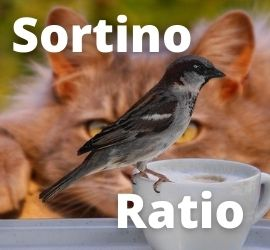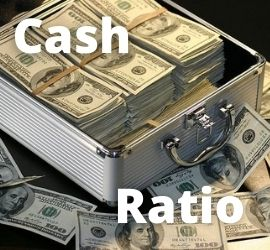# Sortino Ratio: Definition – Analysis – When to Use – Example

## What Is the Sortino Ratio?The Sortino ratio evaluates risk vs return on an investment or portfolio. It is a variation of the Sharpe ratio but it only factors in downside deviation.

For an investor, the ratio helps determine an investment’s return relative to risk. Investors can also use it to differentiate between investments with differing returns and risk profiles.

The Sortino Ratio subtracts a portfolio’s risk-free rate from its return. It then divides that number by the investment or asset’s downside deviation. Downside deviation is a measure of downside risk that considers whether an asset or investment falls below a certain threshold.  As a tool, it essentially helps level the playing field when it comes to assessing risk. Consequently, the Sortino Ratio can help investors determine if an investment’s returns are high enough to consider moving forward.

The Sortino ratio is a variation of the Sharpe ratio.  While the Sharpe ratio considers overall volatility, the Sortino ratio differentiates harmful volatility from total overall volatility.  It does this by using the asset’s standard deviation of negative portfolio returns to only measure downside deviation.  The theory is that upside volatility is a good thing.  As a result, the Sortino ratio ignores the good volatility and only considers the bad volatility which is measured as downside deviation. The ratio was named after Frank A. Sortino.

## Sortino Ratio – A Deeper Look

Many investors tend to concentrate only on the rate of return of an asset or their portfolio. However, it is better to also consider the associated level of risk. Risk refers to the likelihood that an asset’s or security’s financial performance will deviate from what is expected. Downside risk is a potential loss from your investment. Conversely, a potential financial gain is known as an upside risk.

Unfortunately, many performance metrics fail to account for the variation in the risk of an investment. They merely calculate their rates of return. However, the Sortino ratio examines changes in the risk-free rate.  As a result, it enables investors to make more informed decisions.

The Sharpe ratio is another metric that helps individuals gauge the performance of an investment after adjusting for risk. However, the Sortino ratio acknowledges the difference between upside and downward risks. More specifically, it provides an accurate rate of return, given the likelihood of downside risk.  Conversely, the Sharpe ratio treats both upside and downside risks equally.

The formula for calculating the Sortino ratio is:

Sortino Ratio = (Average Realized Return – Expected Rate of Return) / Downside Risk Deviation

### Sr = (Ar – Er) / DR

• Sr – Sortino ratio
• Ar – Average realized return
• Er – The expected rate of return
• DR – Target downside deviation

The average realized return refers to the weighted mean return of all the investments in an individual’s portfolio. On the other hand, the expected rate of return (required return rate), or risk-free rate, is the return on long-term government securities.

## Sortino Ratio – What Can it Tell You?

The Sortino ratio is useful for investors, analysts, and portfolio managers.  It can help to evaluate an investment’s return for a given level of negative risk.  Investors should never focus entirely on rate of return without evaluating the corresponding associated level of risk.  This ratio uses only the downside deviation as its risk measure.  By not using total deviation in the calculation, it addresses the problem of using total risk, or standard deviation.  This is important because upside volatility is beneficial to investors and isn’t a factor most investors worry about.

### What is a good Sortino ratio?

A higher Sortino ratio is better than a lower one.  It indicates that the portfolio is operating efficiently.  It is not taking on the unnecessary risk that is not being rewarded in the form of higher returns. A low, or negative, Sortino ratio may suggest that the investor is not being rewarded for taking on additional risk.

## Sortino Ratio – Example Calculation

When comparing two similar investments, the one with the higher Sortino ratio has an edge.  The higher number means that the investment is earning more return per unit of negative risk that it takes on.

For example, assume Mutual Fund X has an annualized return of 12% and a downside deviation of 10%. Mutual Fund Z has an annualized return of 10% and a downside deviation of 7%. The risk-free rate is 2.5%.  (Note: The expected rate of return (required return rate), or risk-free rate, is the return on long-term government securities.)

The Sortino ratios for both funds is calculated as follows:

### Mutual Fund ABC

• Average realized return 13%
• Risk-free rate of return 2.0%
• Downside deviation 10%

Sortino Ratio (ABC) = (13% – 2.0%) / 10% = 1.10

• Average realized return 11%
• Risk-free rate of return 2.0%
• Downside deviation 8%

Sortino Ratio (XYZ) = (11% – 2.0%) / 8% = 1.125

Mutual Fund ABC returns 2% more on an annualized basis.  However, it is not earning that return as efficiently as Mutual Fund XYZ.  Factoring in their downside deviations, Mutual Fund XYZ is the better choice when taking into account downside risk potential.

While using the risk-free rate of return is common, investors can also use expected returns in calculations. For accuracy, the investor should be consistent in terms of the type of return used in the calculation.

## The Difference Between the Sortino Ratio and the Sharpe Ratio

Both the Sortino and the Sharpe ratios are risk-adjusted evaluations of return on investment.  But, the Sortino ratio improves upon the Sharpe ratio by isolating downside or negative volatility.  The Sharpe ratio measures total volatility by dividing excess return by the downside deviation instead of the total standard deviation of a portfolio or asset.  However, the Sharpe ratio punishes the investment for good risk, which provides positive returns for investors. Ultimately, which ratio to use depends on which measure of volatility matters.  Does the investor want to focus on total or standard deviation, or just downside deviation?

• Sharpe ratio takes a portfolio return, subtracts the risk-free rate, and divides the difference by the investment’s total volatility. This makes it easier to compare different portfolios to see which reward investors the most per unit of risk taken.  The Sharpe Ratio is calculated using the risk-free rate, return, and standard deviation of that asset or portfolio. Standard deviation is the total volatility of an investment. That’s both negative and positive volatility. So yes, even an investment’s unexpected positive returns fall under the measure of standard deviation and lowers the result.
• Sortino ratio is a better measure of risk-adjusted returns.  Upside volatility is what investors should look to target.  Therefore, this type of volatility shouldn’t be seen as bad or negatively affect the equation.  The Sortino Ratio takes into account an asset or portfolio’s risk-free rate, its return, and most importantly, its downside deviation.  As a result, it isolates the amount of negative volatility that a particular asset contains.

## When to Use the Sortino Ratio

Both the Sortino and the Sharpe ratio are used to calculate an investment’s risk-adjusted return.  The difference is solely based on if you want to consider total volatility using the standard deviation or the downside volatility using only the downside deviations. Isolating downside deviation does not penalize an unexpected upside. Financial analysis is not a strict science.  Analysts are free to use their own judgment to decide which calculation best supports their investigation.

Compared to the Sharpe ratio, the Sortino ratio is a superior metric.  It only accounts for the downside variability of risks. Such an analysis makes sense, as it enables investors to focus entirely on downside risks.  Of course, this is what they obviously should worry about. Upward risks aren’t really a cause for concern.  Upward volatility is when an investment generates an unexpected financial gain.  That’s a GOOD thing!

By comparison, the Sharpe ratio treats upside and downside risks in the same way. It means that even those investments that produce gains are penalized, which should not be the case. Therefore, the Sortino ratio should be used to assess the performance of high volatility assets, such as shares. In comparison, the Sharpe ratio is more suitable for analyzing low volatility assets, such as bonds. (Source: corporatefinanceinstitute.com)

### Key Considerations

The Sortino ratio is an excellent metric for comparing risky investments.  However, there are a couple of things to keep in mind and take into account.

• Timeframe – It is most accurate to consider investments made over several years.  At the very least, those made during a complete business cycle. Doing so allows you to account for both positive and negative stock returns. Recording only the positive stock returns realized during an economic upturn is only half the picture.  It is likely not a true reflection of an investment over the long term or a complete cycle.
• Liquidity of the assets – A portfolio can be constructed in ways that make it appear less risky.  But the appearance may be because the underlying assets being held are illiquid. For example, privately-owned companies do not trade stock on the open market,  As a result, they are illiquid. If their stock price was analyzed using a Sortino ratio (or Sharpe ratio), the constant value and lack of volatility might yield risk-adjusted returns that are favorable.  However, in reality, that may not be the case.

## Final Words

Rational investors are inherently risk-averse.  That means they should only take the additional risk if it is compensated by additional return. The Sharpe ratio is a common measure of risk-return trade-off. It compares excess return with the total standard deviation of the portfolio’s investment returns.  But, the total deviation is a measure of both the deviations above the mean return and those below the mean return. Upside deviations are good for an investor.  So, the real risk which investors should worry about is the risk of returns falling below the mean. The Sortino ratio defines risk as to the risk of downside variation only.  As a result, this provides a better picture of risk-adjusted performance than the Sharpe ratio.

The Sortino ratio is almost identical to the Sharpe ratio, but it differs in one key respect. The Sharpe ratio accounts for risk adjustments in investments with both positive and negative returns. In contrast, the Sortino ratio examines risk-adjusted returns, but it only considers the downside risks. As a result, the Sortino ratio is seen as a better indicator of risk-adjusted returns.  It doesn’t penalize upside risks, nor should it.  Upside volatility is a good thing and not a cause for concern to investors.

## Up Next: What Is the Cash Ratio?The cash ratio is a liquidity measure that indicates a company’s ability to pay off short-term debt with its cash and cash equivalents.

The ratio provides a quick glimpse at a company’s liquidity.  Specifically, the ratio of a company’s total cash and equivalents to its current liabilities. The metric lays bare a company’s ability to repay its short-term debt with cash or near-cash resources, for example, marketable securities. This information is useful to creditors when deciding if and how much money they would be willing to loan a company.

The cash ratio compares a company’s cash and other liquid assets to its current liabilities. It is a handy tool to quickly estimate a company’s ability to pay off its short-term debt. It tells creditors and analysts the value of current assets that could quickly be turned into cash.  Also, what percentage of the company’s current liabilities these cash and near-cash assets could cover.